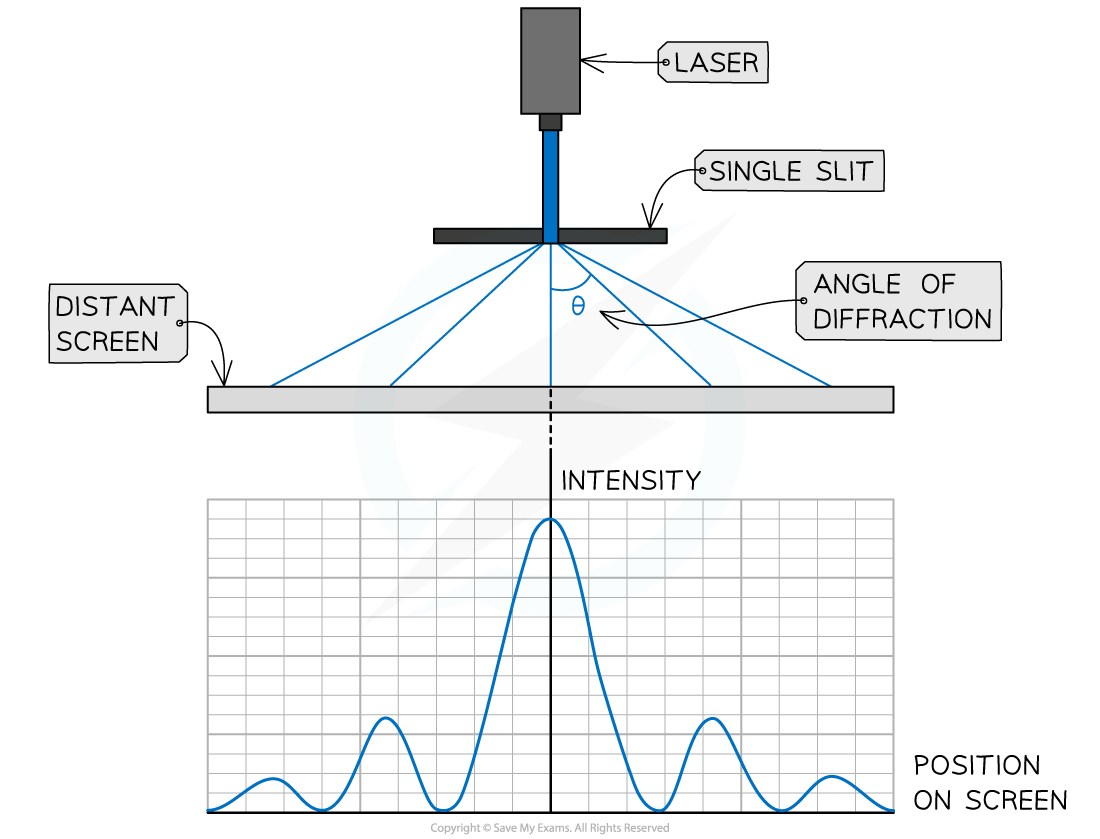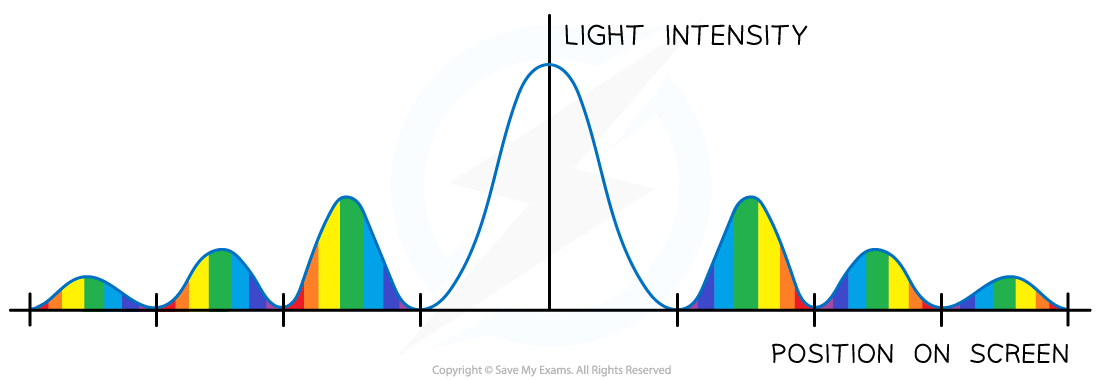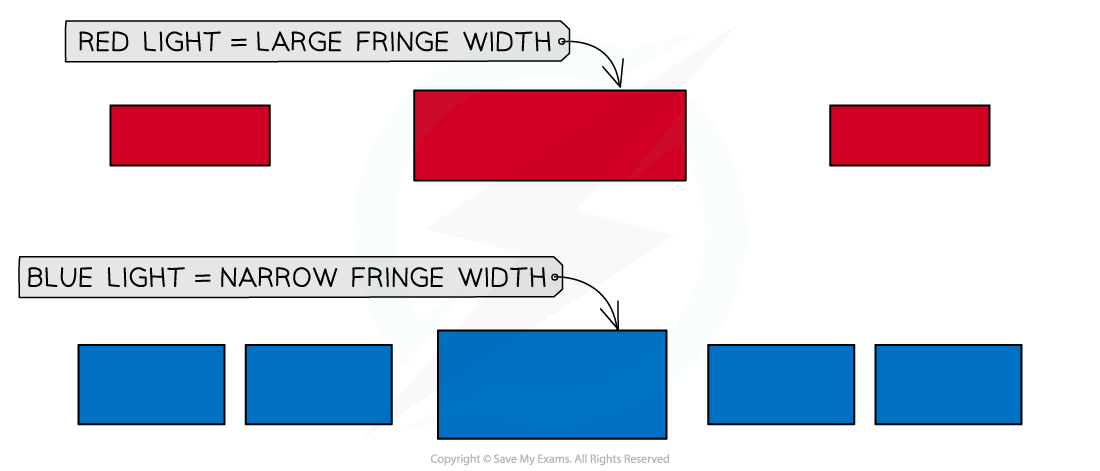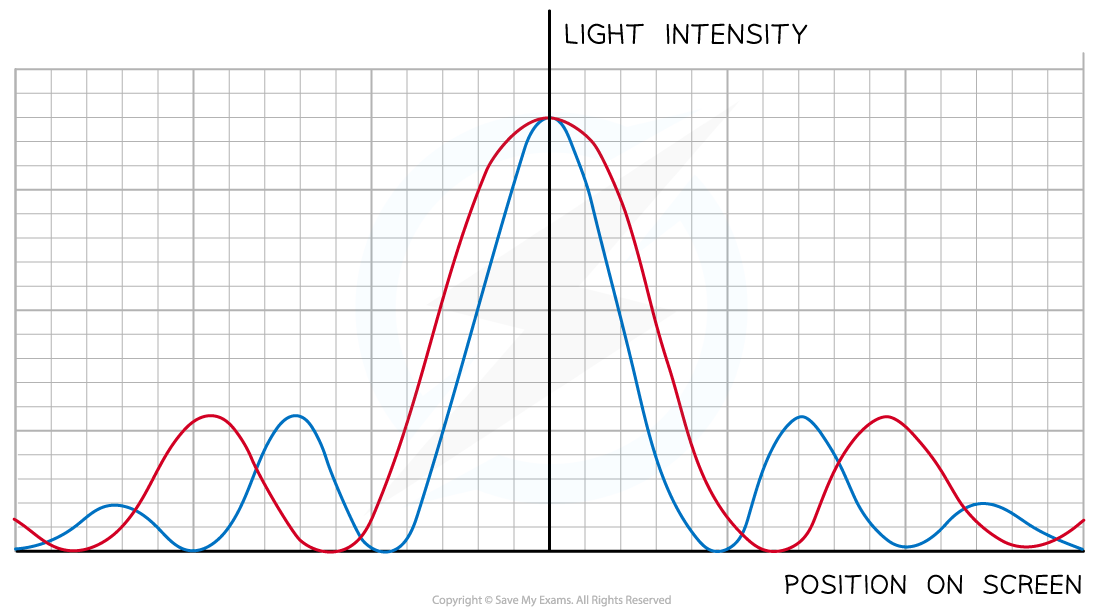# IB DP Physics: HL复习笔记9.2.1 The Nature of Single-Slit Diffraction

### The Nature of Single-Slit Diffraction

• When plane waves are incident normally on a single slit, a diffraction pattern is produced
• This is represented as a series of light and dark fringes which show the areas of maximum and minimum intensity
• If a laser emitting blue light is directed at a single slit, where the slit width is larger than the wavelength of the light, it will spread out as follows:The intensity pattern of blue laser light diffracted through a single slit

• The features of the single-slit diffraction pattern using monochromatic light are:
• A central maximum with a high intensity
• Equally spaced subsidiary maxima, successively smaller in intensity and half the width of the central maximum

#### Single Slit Diffraction of White Light

• When white light is incident on a slit, separate diffraction patterns can be observed for each wavelength making up the white lightSingle slit diffraction of a white light source

• If the laser were to be replaced by a non-laser source emitting white light:
• The central maximum would be white
• All maxima would be composed of a spectrum
• The shortest wavelength (violet / blue) would appear nearest to the central maximum
• The longest wavelength (red) would appear furthest from the central maximum
• The fringe spacing would be smaller and the maxima would be widerQualitative treatment of the variation of the width of the central diffraction maximum with wavelength and slit width. Red light is diffracted the most, blue light is diffracted the least.

#### Exam Tip

Be mindful that all waves undergo diffraction, so questions about diffraction may involve sound, ultrasound, electromagnetic waves, or even waves on water

### Slit Width

• The angle of diffraction is directly proportional to the wavelength of the light
• This means that the width of the bright maxima, or fringe, is also proportional to the wavelength
• Red light – which has the longest wavelength of visible light – will produce a diffraction pattern with wide fringes
• Blue light – which has a much shorter wavelength – will produce a diffraction pattern with narrow fringesFringe width depends on the wavelength of the light

• Therefore, if the blue laser were to be replaced with a red laser:
• The wavelength of red light is longer so the light would diffract more
• The intensity fringes would therefore be widerThe intensity pattern of red laser light shows longer wavelengths diffract more than shorter wavelengths

• If the slit was made narrower:
• The intensity would decrease
• The fringe spacing would be wider

#### Exam Tip

When drawing diffracted waves, take care to keep the wavelength (the distance between each wavefront) constant. It is only the amplitude of the wave that changes when diffracted.